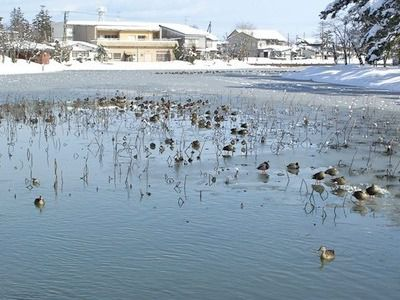# Welcome to Nakagawa's Homepage

## Jin Nakagawa (Number Theory)

I am working in algebraic number theory. In particular, I am interested in the distribution of the discriminants of algebraic number fields in connection with class numbers of binary forms, zeta functions associated with prehomogeneous vector spaces and Igusa's local zeta functions. I intend to apply the results of these research to the study of unramified Galois extensions of algebraic number fields, class numbers of algebraic number fields and Iwasawa theory.

Introduction to Algebraic Number Fields

## Publications

• A conjecture on the zeta functions of pairs of ternary quadratic forms, Amer. J. of Math. 143, 335-410 (2021)
• Class numbers of pairs of symmetric matrices, Acta Arithmetica 105, 207-225 (2002)
• On the relations among the class numbers of binary cubic forms, Invent. math. 134, 101-138 (1998)
• Orders of a quaternion algebra over a number field, J. reine angew. Math. 479, 183-194 (1996)
• Orders of a quartic field, Memoirs Amer. Math. Soc., No. 583 (1996)
• Orders of quadratic extensions of number fields, Acta Arithmetica LXVII, 229-239 (1994)
• Binary forms and unramified $A_n$-extensions of quadratic fields, J. reine angew. Math. 406, 167-178 (1990) (Erratum, ibid. 413, 220 (1991))
• Binary forms and orders of algebraic number fields, Invent. math. 97, 219-235 (1989) (Erratum, ibid. 105, 443 (1991))
• Elliptic curves with no rational points (joint work with K. Horie), Proc. Amer. Math. Soc. 104, 20-24 (1988)
• On the Galois group of a Number field with square free discriminant, Comment. Math. Univ. Sancti Pauli 37, 95-98 (1988)
• Class numbers of quadratic extensions of algebraic number fields, Tohoku Math. J. 38, 245-257 (1986)
• On the Stark-Shintani conjecture and cyclotomic $\mathbb{Z}_p$-extensions of class fields over real quadratic fields II, Tohoku Math. J. 36, 439-452 (1984)
• On the Stark-Shintani conjecture and cyclotomic $\mathbb{Z}_p$-extensions of class fields over real quadratic fields, J. Math. Soc. Japan 36, 577-588 (1984)

## Hobby

My hobby is taking photographs of countryside.